Porosity Resistivity Crossplot (Hingle Plot)
The porosity resistivity crossplot is a venerable tool, still used in many areas. In one version, porosity is plotted on a linear scale, and resistivity on a scale such that straight lines on the graph represent constant water saturation, as determined by the Archie formulae:
1: Sw = (F * RW@FT / RES) ^ (1 / N)
2: F = A / (PHIe ^ M)

Where:
A = tortuosity exponent (fractional)
F = formation factor (fractional)
M = cementation exponent (fractional)
N = saturation exponent (fractional)
PHIe = effective porosity (fractional)
RESD = deep resistivity (ohm-m)
RW@FT = water resistivity (ohm-m)
Sw = water saturation (fractional)

This plot is often called the Hingle plot after the man who first publicized the method. The graph requires a special grid, since the Y axis is linear in the function RESD ^ (-1 / M) but not linear in RESD. RESD or COND lines are used to plot and read data points, so these are plotted to fall non-linearly on the graph paper. The log-log Pickett plot described below is more common today because it is easier to generate with common computer software.

On Hingle plot graph paper  the saturation lines fan out from the zero porosity, infinite resistivity point. The 100% water saturation line can be placed by calculating RESD for any positive value of porosity from the Archie formula. Similarly other saturation lines can be placed on the graph. By rearranging the Archie equation we get:
3: RESD = A * RW@FT / (PHIe ^ M) * (Sw ^ N)

Where:
A = tortuosity exponent (fractional)
F = formation factor (fractional)
M = cementation exponent (fractional)
N = saturation exponent (fractional)
PHIe = effective porosity (fractional)
RESD = deep resistivity (ohm-m)
RW@FT = water resistivity (ohm-m)
Sw = water saturation (fractional)

If we take A = 1.0, M = N = 2.0, PHIe = 0.1 and RW@FT = 0.25, then:
Sw = 1.0, RESD = 0.25 / (0.1 ^ 2) / (1 ^ 2) = 25
Sw = 0.7, RESD = 0.25 / (0.1 ^ 2) / (0.7 ^ 2) = 50
Sw = 0.5, RESD = 0.25 / (0.1 ^ 2) / (0.5 ^ 2) = 100
Sw = 0.2, RESD = 0.25 / (0.1 ^ 2) / (0.25 ^ 2) = 625

Therefore, for this example we would draw a line from the PHIe = 0, RESD = infinity point to a point defined by PHIe = 0.1 and RESD = 25, to obtain the 100% water saturation line. The 50% water saturation line joins the origin with the point PHIe = 0.1 and RESD = 100 and so on, as shown in the illustration at the right.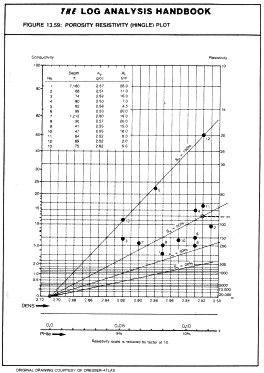If RW@FT is unknown, a line can be drawn slightly above the most northwesterly points on the graph to intersect at the origin and RW@FT back calculated from any point on the line by using:
4: RW@FT = RESD * (PHIe ^ M) / A

Where:
A = tortuosity exponent (fractional)
M = cementation exponent (fractional)
PHIe = effective porosity (fractional)
RESD = deep resistivity (ohm-m)
RW@FT = water resistivity (ohm-m)

If sufficient porosity range exists in the water zone, the northwesterly line can be drawn without knowledge of the porosity origin, thus helping to find the matrix point. In the above illustration, the data suggests a matrix density of 2.7 gm/cc, so the porosity scale origin is set at this point. If data was in porosity units to begin with, this technique would define the matrix offset to correct the porosity log to the actual matrix rock present.

Any of the three porosity logs, (sonic, density, neutron) or any derived porosity, such as density neutron crossplot porosity, can be used for the porosity axis. Any deep resistivity or conductivity reading can be used on the Y axis.

If shallow resistivity data are available, the parameter RESS*RW/RMF can be plotted below the RESD points. The distance between the RESD and normalized RESS points represents the moveable hydrocarbon - the larger the better.

The manual construction of this crossplot can be summarized as follows:
1. Select proper crossplot paper.
2. Scale the X-axis in linear fashion for raw logging parameters (DELT, DENS, PHIN or PHID) and establish porosity scale. Porosity will be zero at the matrix point and increases to the right.
3. Plot resistivity (RESD) vs log data (DELT, DENS, PHIN or PHID). The resistivity scale can be changed by any order of magnitude to fit the log data. This can be done without changing the validity of the graph paper grid.
4. The straight line drawn through the most north westerly points defines Sw = 1.0. Extrapolate this to the intersection with X-axis (PHIe = 0, RESD = infinity).
5. At the intersection, determine the matrix value (DELTMA or DENSMA) for a proper porosity scaling of the X-axis. If logs are in porosity units, this line will determine the matrix offset.
6. Calculate RW@FT from any corresponding pair of PHIe and RESD data along the water line.
7. Determine lines of constant Sw values based on the Archie equation (for any given PHIe value). Keep in mind that all these lines must converge at the matrix point.
8. Read and evaluate Sw values for all points plotted on the crossplot. Make sure points are numbered to avoid confusion, particularly if very long sections are analyzed.
9. As an extension of this method, in case RESS data are also available, the moveable hydrocarbon can be determined by plotting RESD * RW / RMF below each RESD point.

The grid for a Hingle plot is difficult to draw by hand as the resistivity axis is non-linear. Blank forms are available in most service company chart books, as well as here:
Hingle plot M = 2.00 Full Size
Hingle plot M = 2.15 Full Size

Reference:
1. The Use of Logs in Exploration Problems
A.T. Hingle, SEG, 1959Resistivity Porosity Crossplot (Pickett PLOT)
Since the non-linear graph paper of the Hingle plot is difficult to construct, another style of porosity resistivity plot is popular. It is called a Pickett plot, and both resistivity and porosity are plotted on logarithmic scales.

Again, by rearranging the Archie equation we get:
5: log RESD = -M * log PHIe + log (A * RW@FT) - N * log Sw

When Sw = 1.0, then:
6: log RESD = -M * log PHIe + log (A * RW@FT)
7: M = (log(A*RW@FT) - log(RESD)) / log(PHIe)

This is the equation of a straight line on log - log paper. The line has a slope of (-M) and the intercept when PHIe = 1 is the value of A * RW@FT.

If resistivity increases upward and porosity increases to the right, a line drawn slightly below the south westerly data points should represent the 100% water saturation line (as long as a water zone exists in the interval). If A * RW@FT is known, the line should pass through this point at PHIe = 1.0.

If the cementation exponent M is known, the line can be drawn with this slope to find A * RW@FT. Remember that M is seldom less than 1.7 or more than 2.8 in non-fractured reservoirs.

The slope is determined manually by measuring a distance on the RESD axis (in cm. or inches) and dividing it by the corresponding distance on the porosity axis, or by using equation 7. The result will always be negative.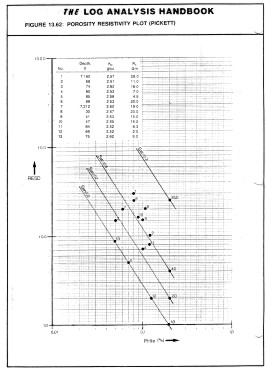To construct the other water saturation lines, first draw a line upward from the point where the 100% water saturation line meets the line RESD = 1.0. Then mark points on the vertical line at RESD values of 2.0, 4.0 and 25.0. Draw a line through each of these marks parallel to the 100% water saturation line. These lines are 70%, 50% and 20% water saturation lines respectively.

An example is shown at right, using the same data as in the Hingle plot shown earlier. Because A and M and the matrix values for rocks are seldom the world wide averages commonly assumed, the porosity resistivity crossplot is often used to find reasonable values prior to or in lieu of special core studies.

If RW@FT varies, this may be noticed by parallel groupings of data belonging to several distinct water zones. The sequence should be zoned to create a separate plot for each different water resistivity value. Comparisons of these plots between wells are often useful. A shift of data in the porosity direction may indicate a mis-calibrated porosity log.

A shift in the resistivity direction may indicate a mis-calibrated resistivity log, differences in invasion, a change in pore geometry, or a change in A * RW@FT. In the example above, the W axis (colour) is coded red for PE near 3.0 and blue for PE near 5.0, thus segregating dolomite from limestone. Note that the porosity distribution and the slope of the line through the red data is different than that through the blue data. This demonstrates that the pore geometry for the dolomite interval is different than that for the limestone. The M value for the dolomite is less than 2.0 for the dolomite and considerably higher than 2.0 for this limestone. (RESD is on the X axis in this plot).

If RW@FT is known from water samples, it may help define the value for A, which varies primarily with grain size and sorting. This is a function of position in the basin and distance from source rock.

Again, as for the Hingle plot, values of RESS * RW / RMF can be plotted to estimate moveable hydrocarbon. If no water zone exists in the interval, plotting RESS vs PHIe may find the slope M, since RESS sees mostly a water filled zone.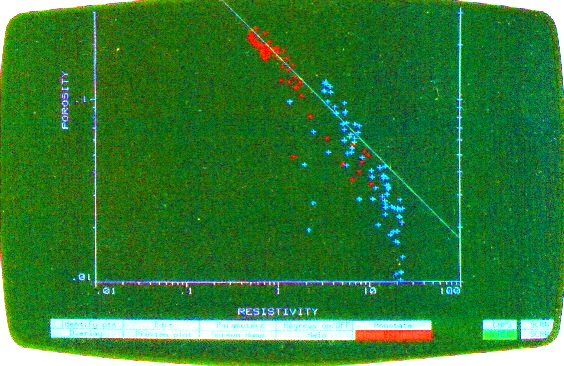Example of Computer-drawn Pickett Plot

The Pickett plot above shows that cementation exponent (M) varies with lithology. The slope of the line through the dolomite data (red) is less than that through the limestone (blue). For a good estimate of water saturation in both zones, the appropriate value of M must be used in each zone. An average line through this data set will make the high porosity dolomite look too wet. The low porosity limestone would appear not wet enough.

Reference:3. Pattern Recognition as a Means of Formation Evaluation
3. Pattern Recognition as a Means of Formation Evaluation
.R. Pickett, SPWLA, 1972Porosity - Water Saturation Crossplot (Buckles Plot)
The product of porosity and minimum water saturation, PHI * SWir, in many rocks is a constant, and the product is called Buckles' Number, after the man who first described this factor:
8: KBUCKL = PHIe * SWir

KBUCKL is found in a clean hydrocarbon bearing zone with a known RW and is used to calculate SWir in depleted reservoirs or in water zones, or in zones of similar rock type with an unknown RW.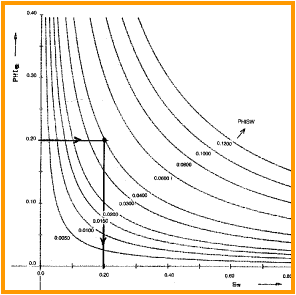It can also be found by plotting core porosity vs minimum wetting phase saturation at an arbitrary capillary pressure from special core analysis data. A graph of this relationship is shown at right. Lower Buckle's Numbers indicate larger average grain size, lower surface area, and lower irreducible water saturation.

Water saturation versus porosity-saturation
product (Buckles Method)

Buckles' equation is used to estimate water saturation by rearranging the terms:
9 : SWp = KBUCKL / PHIe / (1 - Vsh)

If regression is used to determine SW from PHIe, the relationship is usually hyperbolic (KBUCKL = constant) or a skewed hyperbola (KBUCKL varies with porosity).

The shale term has been added by the author to raise KBUCKL and Swb automatically for the finer grained nature of shaly sands.TYPICAL VALUES

Sandstones                  Carbonates              KBUCKL

Very fine grain            Chalky                          0.120

Fine grain                   Cryptocrystalline          0.060

Medium grain             Intercrystalline             0.040

Coarse grain               Sucrosic                       0.020

Conglomerate             Fine vuggy                   0.010

Unconsolidated           Coarse vuggy               0.005

Fractured                    Fractured                     0.001
Gas Shale                                                    0.009 - 0.025

Use these parameters only if no other source exists.COMBINING Porosity - Water Saturation Crossplots
Combining a Pickett Plot and a Buckles Plot on the same graph gives some interesting results. The following is from Dr. Gene Ballay's "Double Duty" newsletter article (www.geoneurale.com).

Reservoir performance can often be evaluated in terms of the Bulk Volume Water BVW = PHI * SW. Contour lines of constant bulk volume water may be used as cut­off boundaries. Permeability estimates may also be possible in favorable situations.  The graphic consists of Water Saturation versus Porosity. Depending upon local conventions, either attribute (porosity or water saturation may be  along the vertical axis, with the other being along the horizontal. In the Log­Log world (such as used in a Pickett Plot), these BVW trends are straight lines, as illustrated on the Buckles Plots shown below.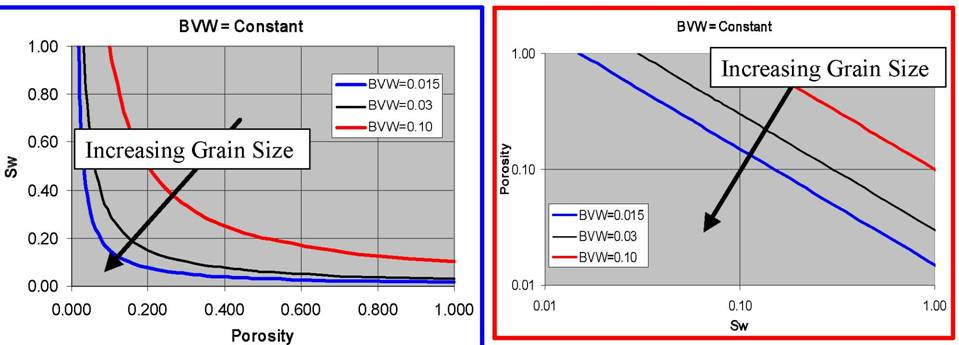Linear axis Buckles Plot of PHI * SW (left) and log-log plot of same  (right). Hyperbolic PHI * SW lines become straight lines in the log-log domain.

On a Pickett Plot, points of constant water saturation will plot on a straight line with slope related to cementation exponent M. Saturation exponent N determines the separation of the Sw = constant grids, as shown below. A*Rw@FT can be deduced from the intercept of the 100% SW line with the 100% porosity lines. The same technique can be applied to the flushed zones, using flushed zone measurements.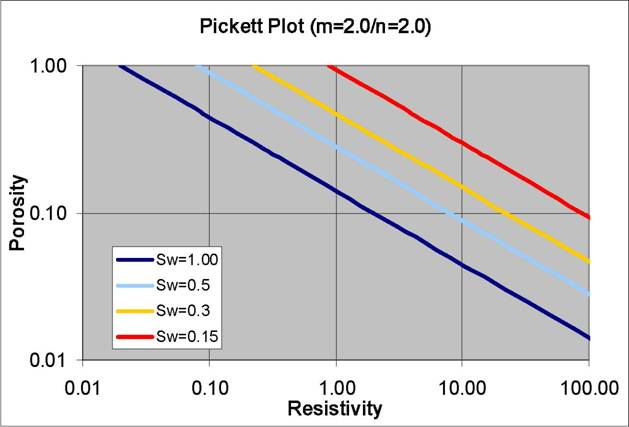Picket Plot on log-log grid for M = N = 2.00.

The full Archie equation:
log Rt = -M * log PHI + log (A * RW@FT) - N * log SW
can be rearranged when M = N to give:

log Rt = log Rw - N * log (PHI * SW) = Constant

Thus vertical lines on a Pickett Plot represent constant PHI * SW (Buckle's Numbers) when M = N.Pickett Plot with constant PHI * SW lines (vertical lines when M = N)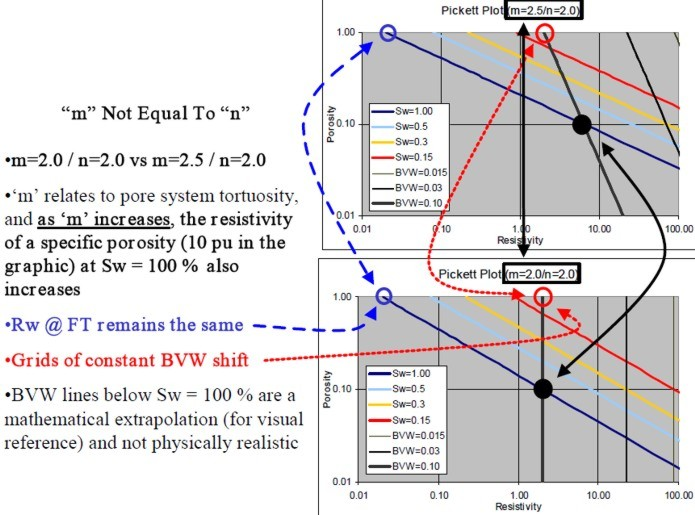Pickett Plot with constant PHI * SW lines when M > NPickett Plot with constant PHI * SW lines when M < N

Reference:
1. Correlating and Averaging Connate Water Saturation Data
R.S. Buckles, CIM, 1965EXAMPLES
These examples of linear axis porosity versus water saturation plots show three different situations in three different wells. First is a well with no water zone and irreducible water that varies along a single hyperbolic trend (constant PHI * SW). The second illustrates a pay zone at constant PHI * SW underlain by a water zone in which PHI * SW varies with porosity. The third example illustrates the need to see the production history and perforated interval data. The well shows increasing SW with depth, as well as increasing PHI * SW with depth. But the well is perforated over the entire interval and produces very little water, so these changes are related to pore geometry, and not a transition zone between water and oil.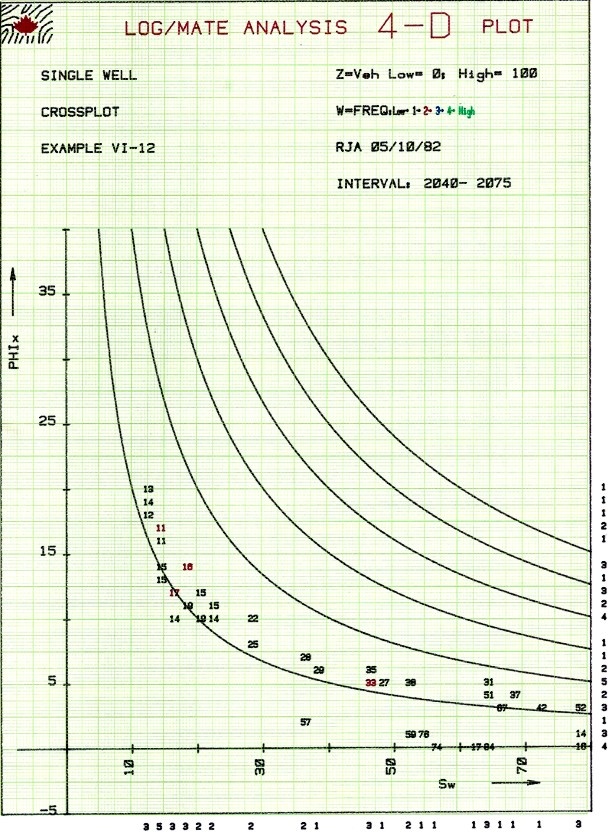Example of a 4-dimensional Buckles Plot. X-axis  = Sw, Y-axis = PHIe, Z-axis (numbers) = Vsh, W-axis (colour) = frequency of occurrence. Hyperbolic lines are constant Buckles Number lines (PHIe*Sw = 0.02, 0.04, 0.06, 0.08, 0.10, 0.12). Numbers along bottom and right edge are histograms of frequency of occurrence. All data points follow near the KBUCKL = 0.025 line and no data points fall to the right side, so this interval will produce no water upon initial production.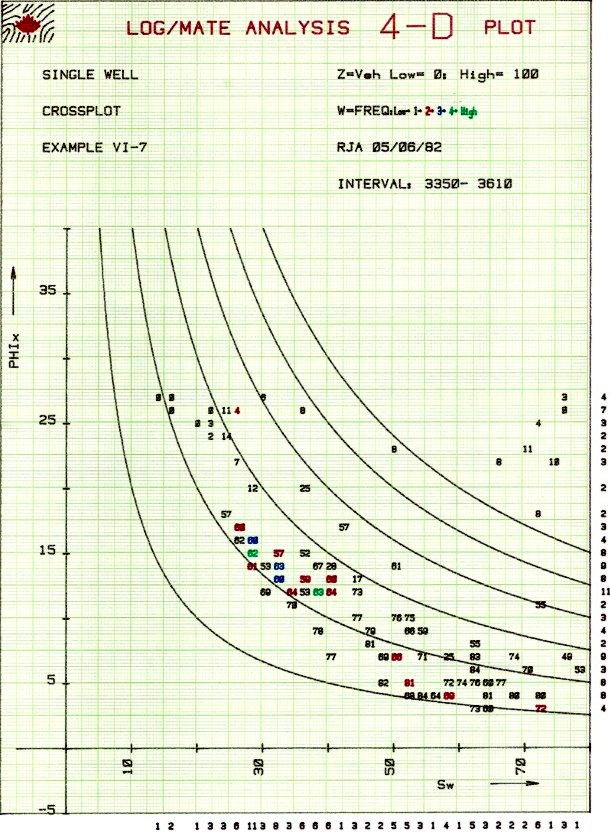Another example in which most data falls along the KBUCKL = 0.02 to 0.04 lines. These points will produce no water. Data points at the far right are from the water zone and will produce water. X, Y, Z, and W axes are as above.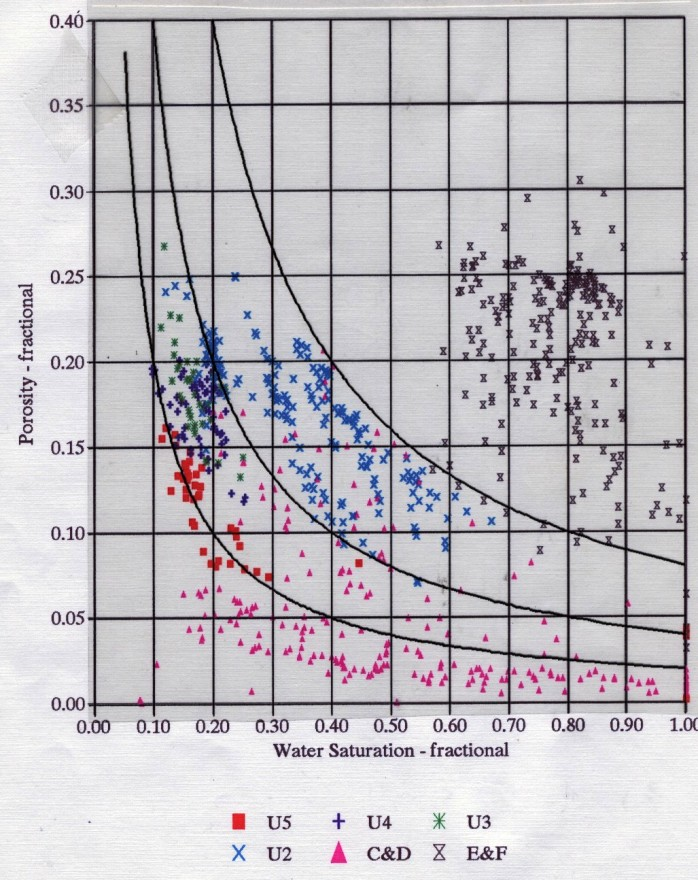Porosity vs water saturation plot in a reservoir with varying pore geometry. Most of these data
points produce clean oil with no water, except the X's on the far right, past Sw = 90%.

Page Views ---- Since 01 Jan 2015
Copyright 2023 by Accessible Petrophysics Ltd.
CPH Logo, "CPH", "CPH Gold Member", "CPH Platinum Member", "Crain's Rules", "Meta/Log", "Computer-Ready-Math", "Petro/Fusion Scripts" are Trademarks of the Author